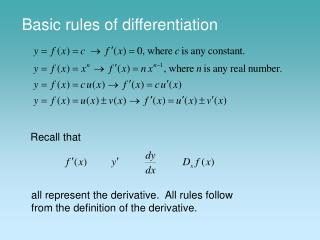Download PresentationBasic rules of differentiation

# Basic rules of differentiation - PowerPoint PPT PresentationDownload Presentation## Basic rules of differentiation

- - - - - - - - - - - - - - - - - - - - - - - - - - - E N D - - - - - - - - - - - - - - - - - - - - - - - - - - -
##### Presentation Transcript

1. Basic rules of differentiation Recall that all represent the derivative. All rules follow from the definition of the derivative.

2. Derivative of a constant Examples

3. Power rule n is any real number Not easy to prove in general. For

4. n is any real number Examples

5. Sum or difference rule Also applies to the sum and difference of more than two functions. Examples

6. Problem 1 The position of an object (in feet) at time t (in seconds) is 1. Find the velocity. 2. Find the velocity at t = 1 and t = 2. 3. Find the time when the velocity is zero. The velocity is zero at t = 3.

7. Sales of digital cameras According to projections made in 2004, the worldwide shipment of digital point-and-shoot cameras are expected to grow in accordance with the rule: where N(t) is measured in millions and t is measured in years, with t = 1 corresponding to 2001. a. How many digital cameras were sold in 2001? 16.3 million digital cameras were sold in 2001

8. b. How fast were sales increasing in 2001? Increasing at the rate of 14.3 million cameras per year c. What were the projected sales in 2005? 66.8 million cameras d. How fast were sales increasing in 2005? Increasing at the rate of 11.7 million cameras per year